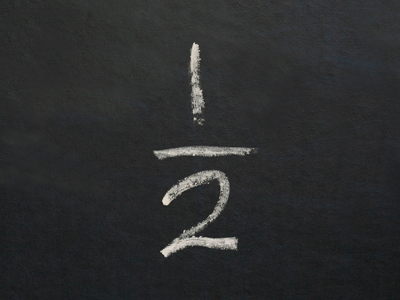# Fractions 6

This Math quiz is called 'Fractions 6' and it has been written by teachers to help you if you are studying the subject at elementary school. Playing educational quizzes is an enjoyable way to learn if you are in the 3rd, 4th or 5th grade - aged 8 to 11.

It costs only \$12.50 per month to play this quiz and over 3,500 others that help you with your school work. You can subscribe on the page at Join Us

Have you heard of 'improper fractions' and 'mixed fractions'? An improper fraction is a fraction in which the numerator is greater than or equal to the denominator, e.g. 53 and 99. A mixed fraction (also called a mixed number) is a number that has a whole number part and a fractional part, which is not written as a decimal, written as a single unit, e.g. 235. Here are a couple of tips:

TIP 1: Don't forget: the numerator is the number at the top and the denominator is the number at the bottom of a fraction.

TIP 2: To convert a mixed fraction to an improper fraction, follow these steps:

1. Multiply the whole number part by the denominator.
2. Add this result to the numerator.
3. Write the fraction with step 2 in the numerator and keep the original denominator.
1.
If you are asked to find 18 of something, what is the best method of doing it?
Subtract 8
Multiply by 8
Divide by 8
The word 'of' means multiply; therefore, one-eighth OF something means multiply by 18 BUT multiplying by an eighth is the same as dividing by eight because 18 × 32 = 328. The same applies in similar situations
2.
What is the improper fraction 165 as a mixed fraction?
3165
315
1615
1065
To convert an improper fraction to a mixed fraction, follow these steps: 1. Divide the numerator by the denominator. 2. Note the whole number remainder. 3. Write the number from step 1 as the whole number in front of the fractional part AND write the fractional part with the remainder in the numerator and keep the original denominator. STEP 1: 16 ÷ 5 = 3. STEP 2: Remainder 1. STEP 3: 315
3.
What is 5418 as a mixed fraction?
3318
3118
113
3
5418 = 3: REDUCE the fraction to its simplest form: divide the numerator and the denominator by 18. Be on the LOOKOUT for this sort of thing. Note: we could have written 311 but this is pointless
4.
Mary says that you can have one fifth of her candies. If she has 125 candies, how many candies will you get?
75
25
45
65
The word 'of' means multiply; therefore one-fifth OF something means multiply by 15 BUT multiplying by a fifth is the same as dividing by five (see question 3): 125 ÷ 5 = 25
5.
What is 335 as an improper fraction?
455
93
185
183
To convert a mixed fraction to an improper fraction, follow these steps: 1. Multiply the whole number part by the denominator. 2. Add this result to the numerator. 3. Write the fraction with step 2 in the numerator and keep the original denominator. STEP 1: 3 × 5 = 15. STEP 2: 15 + 3 = 18. STEP 3: 185
6.
First, Anne had 416 of the cake. Then, James had 916 and finally, Henry had 616 of the cake. Is there anything wrong here?
Anne should have been given more cake because she is a girl
No. Bon appétit!
Yes. Henry didn't get the stated amount of cake
Yes. there won't be any cake left for Henry
416 + 916+ 616 = 1916 which is more than 1 cake! In fact, Henry only got 316 of the cake, which was less than Anne got
7.
Harry lost three-quarters of half the money his aunt had given him. If half of the money was £64, how much did Harry lose?
£96
£32
£24
£48
34 × £64 = (3 × £64) ÷ 4 = 192 ÷ 4 = £48
8.
What is 12 of 14?
34
18
116
132
12 × 14 = 18. You multiply the numbers in the denominator together, and you multiply the numbers in the numerator together to form a single fraction
9.
What is 639 as an improper fraction?
193
189
543
573
To convert a mixed fraction to an improper fraction, follow these steps: 1. Multiply the whole number part by the denominator. 2. Add this result to the numerator. 3. Write the fraction with step 2 in the numerator and keep the original denominator. STEP 1: 6 × 9 = 54. STEP 2: 54 + 3 = 57. STEP 3: 579 BUT you must now REDUCE the fraction to its simplest form: divide the numerator and the denominator by 3 to get 193
10.
What is one seventh of 49?
42
56
7
343
17 × 49 = 49 ÷ 7 = 7
Author:  Frank Evans